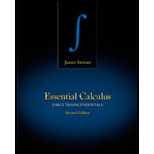# To state: The function of two variables.### Essential Calculus: Early Transcen...

2nd Edition
James Stewart
Publisher: Cengage Learning
ISBN: 9781133112280### Essential Calculus: Early Transcen...

2nd Edition
James Stewart
Publisher: Cengage Learning
ISBN: 9781133112280

#### Solutions

Chapter 11, Problem 1RCC

(a)

To determine

## To state: The function of two variables.

Expert Solution

### Explanation of Solution

Let the function be f(x,y) .

The function of two variables is assigned by a two real numbers in 2 that should be inside the domain region.

Thus, the result obtained is a real number for the region {f(x,y)|(x,y)D} .

(b)

To determine

Expert Solution

### Explanation of Solution

Statement:

Let the function be f(x,y) .

The methods for the function to observe the result is by using graph, vector diagram and level curves.

### Want to see more full solutions like this?

Subscribe now to access step-by-step solutions to millions of textbook problems written by subject matter experts!

### Want to see more full solutions like this?

Subscribe now to access step-by-step solutions to millions of textbook problems written by subject matter experts!